## 1 Introduction

In many formal methods applications, such as verification, it is common to represent proof obligations in terms of the Satisfiability Modulo Theories (SMT) problem. SMT solvers have thus become popular backends for such applications. They have been primarily designed to be decision procedures for quantifier-free problems, on which they are highly efficient and capable of handling large formulas over background theories. Quantified formulas are generally handled with instantiation techniques that are often incomplete, even on decidable or semi-decidable fragments. Heavily relying on incomplete heuristics however leads to instability and unpredictability on the solver’s behavior, which is undesirable for the tools relying on them. To address these issues some systems use model-based instantiation (MBQI) , a complete technique for first-order logic with equality and for several restricted fragments containing theories, which can be used as a fallback strategy to the incomplete techniques.

In this paper we introduce a novel enumerative instantiation technique which can serve as a simpler alternative to model-based instantiation. Similar to MBQI, our technique can be used as a secondary strategy when incomplete techniques fail. Our experiments show that a careful implementation of this technique in the state-of-the-art SMT solver CVC4 leads to noticeable gains in performance on unsatisfiable problems.

Background. Some of the earliest tools for theorem proving in first-order logic come from the work by Skolem and Herbrand. The Herbrand Theorem states that if a closed formula in Skolem normal form, i.e. a prenex formula without existential quantifiers, is unsatisfiable, then there is an unsatisfiable finite conjunction of Herbrand instances of the formula, that is, instances on terms from the Herbrand universe, i.e. the set of all possible well-sorted ground terms in the formula’s signature. The first theorem provers for first-order logic to be implemented based on Herbrand’s theorem employed a completely unguided search on the Herbrand Universe (e.g. Gilmore  and Davis et al.  early efforts). Such systems were only capable of dealing with very simple formulas and were soon put aside. Techniques which would only generate Herbrand instances when needed were first introduced by Prawitz  and later refined by Davis and Putnam , culminating in the resolution calculus introduced by Robinson . The most successful techniques for handling pure first-order logic have been based on resolution and ordering criteria . More recently, techniques based on instantiation have shown promise for first-order logic as well [13, 17, 28]. Inspired by early work on the subject, this paper revisits whether modern implementations of the latter class of techniques can benefit from enumerative instantiation.

Outline. We first give preliminaries in Sect. 2. Then, we introduce a stronger Herbrand Theorem as the basis for making enumerative instantiation practical so that it can be used in modern systems in Sect. 3. We formalize the different instantiation strategies used by state-of-the-art SMT solvers, discuss their strengths and weaknesses, and present a schematization of how to combine such strategies in Sect. 4, with a focus on a new strategy for enumerative instantiation. An extensive experimental evaluation of enumerative instantiation as implemented in CVC4 is presented in Sect. 5.

## 2 Preliminaries

We work in the context of many-sorted first-order logic with equality (see e.g. ) and assume the reader is familiar with the notions of signature, term, (quantified and ground) formula, atom, literal, free and bound variable, and substitution.

We consider signatures $$\varSigma$$ containing a Bool sort and constants $$\top ,\,\bot$$ and a family of predicate symbols $$({\approx } : \tau \times \tau \rightarrow \mathsf {Bool})$$ interpreted as equality for each sort $$\tau$$. Without loss of generality, we assume $$\approx$$ is the only predicate in $$\varSigma$$. We use $$=$$ for syntactic equality. The set of all terms occurring in a formula $$\varphi$$ (resp. term t) is denoted by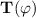(resp.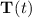). We write $$\bar{t}$$ for the sequence of terms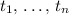for an unspecified $$n \in \mathbb {N}^+$$ that is either irrelevant or deducible from the context.

An interpretation is a triple $$\mathscr {M}=(\mathscr {D},\,\mathscr {I},\,\mathscr {V})$$ in which $$\mathscr {D}$$ is a collection of non-empty domain sets for all sorts in $$\varSigma$$, $$\mathscr {I}$$ interprets symbols by mapping them into functions over domain sets according to the symbol sort, and $$\mathscr {V}$$ maps free variables to elements of their respective domain sets. A theory is a pair $$\mathscr {T}=(\varSigma ,\,\varOmega )$$ in which $$\varSigma$$ is a signature and $$\varOmega$$ is a class of interpretations denoted the models of $$\mathscr {T}$$. The empty theory is the theory for which the class of interpretations $$\varOmega$$ is unrestricted, which coincides with first-order logic with equality. Throughout this paper we assume a fixed background theory $$\mathscr {T}$$, which unless otherwise stated is the empty theory. A formula $$\varphi$$ is satisfiable (resp. unsatisfiable) in $$\mathscr {T}$$ if it is satisfied by some (resp. no) interpretation $$\mathscr {M}\in \varOmega$$, written $$\mathscr {M}\,\models _{\mathscr {T}}\varphi$$. A formula $$\varphi$$ entails in $$\mathscr {T}$$ a formula $$\psi$$, written $$\varphi \,\models _{\mathscr {T}}\psi$$, if every interpretations in $$\varOmega$$ satisfying $$\varphi$$ also satisfies $$\psi$$. For these notions of model satisfaction and entailment in the empty theory, we omit the subscript.

A substitution $$\sigma$$ maps variables to terms and its domain, $$\mathsf {dom}( \sigma )$$, is finite. We write $$\mathsf {ran}( \sigma )$$ to denote its range. Throughout the paper, conjunctions may be written as sets or tuples, and vice-versa, whenever convenient and unambiguous. All definitions are assumed to be lifted in the expected way from formulas into sets or tuples of formulas.

Instantiation-Based SMT Solvers

Quantifiers in formulas are generally handled by SMT solvers through instantiation-based techniques, which capitalize on their capability to handle large ground formulas. In this approach, an input formula $$\psi$$ is given to the ground SMT solver, which will abstract all atoms and quantified formulas and treat them as if they were propositional variables. The solver for ground formulas will provide an assignment $$\mathsf {E}\cup \mathsf {Q}$$, where $$\mathsf {E}$$ is a set of ground literals and $$\mathsf {Q}$$ is a set of quantified formulas appearing in $$\psi$$, such that $$\mathsf {E}\cup \mathsf {Q}$$ propositionally entails $$\psi$$. We assume that all quantified formulas in $$\psi$$ are of the form $$\forall \bar{x}.\>\varphi$$ with $$\varphi$$ quantifier-free. This can be achieved by prenex form transformation and Skolemization. The instantiation module of the solver will then generate new ground formulas of the form $$\forall \bar{x}.\>\varphi \Rightarrow \varphi \sigma$$ where $$\forall \bar{x}.\>\varphi$$ is a quantified formula in $$\mathsf {Q}$$ and $$\sigma$$ is a substitution from the variables in $$\varphi$$ to ground terms. These instances will be added conjunctively to the input of the ground solver, hence refining its knowledge of the quantified formulas. The ground solver may then provide another assignment $$\mathsf {E}'\cup \mathsf {Q}'$$, where this is a set that entails both $$\varphi$$ and the newly added instances. This new assignment might either be the previous one, augmented by new ground literals coming from the new instances, or if the previous $$\mathsf {E}$$ has been refuted by the new instances, a completely different set. On the other hand, the process may terminate if the newly added instances suffice to prove the unsatisfiability of the original formula. We will refer to the game between the ground solver that provides assignments for the abstraction of the formula and the instantiation module that provides instances added conjunctively to the formula, as the instantiation loop of the SMT solver (see Fig. 1).

## 3 Herbrand Theorem and Beyond

The Herbrand Theorem (see e.g. ) for pure first-order logic with equalityFootnote 1 provides a refutationally complete procedure to check the satisfiability of a formula $$\psi$$, or more specifically of a set of literals and quantifiers $$\mathsf {E}\cup \mathsf {Q}$$. Indeed, $$\mathsf {E}\cup \mathsf {Q}$$ is satisfiable if and only if $$\mathsf {E}\cup \mathsf {Q}_g$$ is satisfiable, where $$\mathsf {Q}_g$$ is the set of all (Herbrand) instances one can build from the quantifiers in $$\mathsf {Q}$$ by instantiation with the Herbrand universe, i.e. all the possible well-sorted terms built on the signature used in $$\mathsf {E}\cup \mathsf {Q}$$. Based on this, an instantiation module has a simple refutationally complete strategy for pure first-order logic with equality: it suffices to enumerate Herbrand instances. The major drawback of this strategy is that the Herbrand universe is large. For instance, as soon as there is a function with the range sort also used as an argument, the Herbrand universe is infinite.

Fortunately, a stronger variant of the Herbrand Theorem holds. Using this variant, the instantiation module does not need to consider all possible well-sorted terms (i.e. the full Herbrand universe), but only the terms already available in $$\mathsf {E}\cup \mathsf {Q}$$, and those subsequently generated.

### Theorem 1

Consider the conjunctive sets $$\mathsf {E}$$ and $$\mathsf {Q}$$ of ground literals and universally quantified clauses respectively where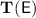contains at least one term of each sort. The set $$\mathsf {E}\cup \mathsf {Q}$$ is unsatisfiable in pure first-order logic if and only if there exists a series $$\mathsf {Q}_i$$ of finite sets of instances of $$\mathsf {Q}$$ such that

• for some number n, the finite set of formulas $$\mathsf {E}\cup \bigcup _{i=1}^{n}\mathsf {Q}_i$$ is unsatisfiable;

•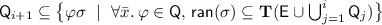.

### Proof

All proofs for this section are included in .    $$\square$$

The above theorem is stronger than the classical Herbrand theorem in the sense that the set of instances considered above is smaller (or equal) than the set of instances considered in the classical Herbrand theorem. As a trivial example, if a function f appears only in $$\mathsf {E}\cup \mathsf {Q}$$ in ground terms, no new applications of f are considered. The theorem does not consider all arbitrary terms from the signature, but only those that are generated by the successive instantiations with only already available ground terms. Note the theorem holds for pure first-order logic with equality, and in any theory that preserves the compactness property. It is also necessary however to consider the axioms of the theory for the generation of new terms, that might lead to other instances.

In the Bernays-Schönfinkel-Ramsey fragment of first-order logic (also know as the EPR class) formulas do not contain non constant function symbols, therefore the Herbrand universe of any formula is a finite set. Since the above sets of terms are a subset of the Herbrand universe, the enumeration will always terminate, even when the formula is satisfiable. Therefore, the resulting ground problem is decidable, and the above method comprises a decision procedure for this fragment, just like some variant of model-based quantifier instantiation.

Theorem 1 implies that an instantiation module only has to consider terms occurring within assignments, and not all possible terms. To show refutational completeness (termination on unsatisfiable input) and model soundness (termination without declaring unsatisfiability implies that the input is satisfiable), it is however necessary to account for the successive assignments produced by the ground SMT solver and the consecutive generation of instances. This is achieved using the following lemma.

### Lemma 1

Consider the conjunctive sets $$\mathsf {E}$$ and $$\mathsf {Q}$$ of ground literals and universally quantified clauses respectively wherecontains at least one term of each sort. If there exists an infinite series of finite satisfiable sets of ground literals $$\mathsf {E}_i$$ and of finite sets of ground instances $$\mathsf {Q}_i$$ of $$\mathsf {Q}$$ such that

•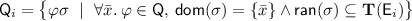;

• $$\mathsf {E}_0 = \mathsf {E}$$, $$\mathsf {E}_{i+1}\,\models \,\mathsf {E}_i \cup \mathsf {Q}_{i}$$;

then $$\mathsf {E}\cup \mathsf {Q}$$ is satisfiable in the empty theory with equality.

The above lemma has two direct consequences on the instantiation loop of SMT solvers, where instances are generated from the set of available terms in the ground assignment provided by the ground SMT solver. The following two corollaries state the model soundness and the refutational completeness of the instantiation loop respectively.

### Corollary 1

Given a formula $$\psi$$, if there exists a satisfiable set of literals $$\mathsf {E}$$ and a set of quantified clauses $$\mathsf {Q}$$ such that $$\mathsf {E}\cup \mathsf {Q}\,\models \,\psi$$ and the instantiation module of the SMT solver cannot generate any new instance, i.e. $$\mathsf {E}$$ already entails all instances of $$\mathsf {Q}$$ for substitutions built with terms, then $$\psi$$ is satisfiable.

### Proof

A formal statement of the corollary and a proof is available in .    $$\square$$

### Corollary 2

Given an unsatisfiable formula, if the generation of instances is fair the instantiation loop of the SMT solver terminates.

### Proof

A formal statement of the corollary and a proof is available in .    $$\square$$

## 4 Quantifier Instantiation in CDCL($$\mathscr {T}$$)

This section overviews recent techniques used by SMT solvers for quantifier instantiation, and comments on their relative strengths and weaknesses. We will focus on enumerative quantifier instantiation, a technique which has received little attention in recent work, but has several compelling advantages with respect to current techniques.

### Definition 1

(Instantiation Strategy). An instantiation strategy takes as input:

1. 1.

A $$\mathscr {T}$$-satisfiable set of ground literals $$\mathsf {E}$$, and

2. 2.

A quantified formula $$\forall \bar{x}.\>\varphi$$.

It outputs a set of substitutions $$\{ \sigma _1, \ldots , \sigma _n \}$$ where $$\mathsf {dom}( \sigma _i ) = \bar{x}$$  for each $$i = 1,\ldots ,n$$.

Figure 2 gives four instantiation strategies used by modern SMT solvers, each that have the interface given in Definition 1. The first three have been described in detail in previous works (see  for a recent overview). We briefly review these techniques in this section. The fourth, enumerative quantifier instantiation, is the subject of this paper.

Conflict-based instantiation ($$\mathbf{c}$$) was introduced in  as a technique for improving the performance of SMT solvers for unsatisfiable problems. In this strategy, we return a substitution $$\sigma$$ such that $$\varphi \sigma$$ together with $$\mathsf {E}$$ is unsatisfiable, We refer to $$\varphi \sigma$$ as a conflicting instance (for $$\mathsf {E}$$). Typical implementations of this strategy do not insist that a conflicting instance be returned if one exists, and hence the strategy may choose to return the empty set of substitutions. Recent work [4, 5] gives a strategy for conflict-based instantiation that has refutational completeness guarantees for the empty theory with equality, that is, when a conflict instance exists for a quantified formula in this theory, the strategy is guaranteed to return it.

E-matching instantiation ($$\mathbf{e}$$) is the most commonly used strategy for quantifier instantiation in modern SMT solvers [13, 15, 18]. In this strategy, we first heuristically choose a set of triggers for a quantified formula $$\forall \bar{x}.\> \varphi$$, where a trigger is a tuple of terms whose free variables are $$\bar{x}$$. In practice, triggers can be selected using user-provided annotations, or selected automatically by the SMT solver. For each trigger $$\bar{t}_i$$, we select a set of substitutions $$S_i$$ such that for each $$\sigma$$ in this set, $$\mathsf {E}$$ entails that $$\bar{t}_i \sigma$$ is equal to a tuple of ground terms $$g_i$$ in $$\mathsf {E}$$. We return the union of these sets $$S_i$$ for each selected trigger. E-matching instantiation is generally incomplete, but works well in practice for unsatisfiable problems, and hence is a key component of most SMT solvers that support quantified formulas.

Model-based quantifier instantiation ($$\mathbf{m}$$) was introduced in , and has also been used for improving the performance of finite model finding . In this strategy, we first construct a model $$\mathscr {M}$$ for the quantifier-free portion of our input $$\mathsf {E}$$, where typically the interpretations of functions for values not constrained by $$\mathsf {E}$$ are chosen heuristically. Notice that $$\mathscr {M}$$ does not necessarily satisfy the quantified formula $$\forall \bar{x}.\> \varphi$$. If it does not, we return a single substitution $$\sigma$$ for which $$\mathscr {M}$$ does not satisfy $$\varphi \sigma$$, where typically $$\sigma$$ maps variables from $$\bar{x}$$ to terms that occur in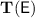. With respect to conflict-based and E-matching instantiation, model-based quantifier instantiation has the advantage that it is model sound: when it returns $$\emptyset$$, then $$\mathsf {E}\cup \{ \forall \bar{x}.\> \varphi \}$$ is satisfiable.

This paper revisits enumerative quantifier instantiation ($$\mathbf{u}$$) as a viable alternative to model-based quantifier instantiation. In this strategy, we assume an ordering $$\preceq$$ on quantifier-free terms. This ordering is not related to the usual term ordering one generally uses for saturation theorem proving, but rather determines which instance will be generated first. The strategy returns the substitution $$\{ \bar{x} \mapsto \bar{t} \}$$, where $$\bar{t}$$ is the minimal tuple of terms with respect to $$\preceq$$ fromsuch that $$\varphi \{ \bar{x} \mapsto \bar{t} \}$$ is not entailed by $$\mathsf {E}$$. We refer to this strategy as enumerative instantiation since in the worst case it generates instantiations by enumerating tuples of all terms of the proper sort from $$\mathsf {E}$$, according to the ordering $$\preceq$$. In practice, the number of instantiations produced by this strategy is kept small by interleaving it with other strategies like $$\mathbf{c}$$ or $$\mathbf{e}$$, or due to the fact that a small number of instances may already allow the SMT solver to conclude the input is unsatisfiable. Moreover, thanks to the results in Sect. 3, this strategy is refutationally complete and model sound for quantified formulas in the empty theory with equality.

### Example 1

Consider the set of ground literals $$\mathsf {E}= \{ \lnot P( a ), \lnot P( b ), P( c ), \lnot R( b ) \}$$. For the input $$( \mathsf {E}, \forall x.\>P( x ) \vee R( x ) )$$, the strategies in this section will do the following.

1. 1.

Conflict based: Since $$\mathsf {E},\, P( b ) \vee R( b )\,\models \,\bot$$, this strategy will return $$\{ \{ x \mapsto b \} \}$$.

2. 2.

E-matching: This strategy may choose the singleton set of triggers $$\{ ( P( x ) ) \}$$. Based on this trigger, since $$\mathsf {E}\,\models \,P( x ) \{ x \mapsto t \} \approx P( t )$$ wherefor $$t = a, b, c$$, this strategy may return $$\{ \{ x \mapsto a \},\, \{ x \mapsto b \},\, \{ x \mapsto c \} \}$$.

3. 3.

Model-based: This strategy will construct a model $$\mathscr {M}$$ for $$\mathsf {E}$$, where assume that $$P^\mathscr {M}= \lambda x.\> \mathsf {ite}( x \approx c,\, \top ,\, \bot )$$ and $$R^\mathscr {M}= \lambda x.\> \bot$$. Since $$\mathscr {M}$$ does not satisfy $$P( a ) \vee R( a )$$, this strategy may return $$\{ \{ x \mapsto a \} \}$$.

4. 4.

Enumerative instantiation: This strategy chooses an ordering on tuples of terms, say the lexicographic extension of $$\preceq$$ where $$a \prec b \prec c$$. Since $$\mathsf {E}$$ does not entail $$P( a ) \vee R( a )$$, this strategy returns $$\{ \{ x \mapsto a \} \}$$.    $$\square$$

In the previous example, clearly $$\{ x \mapsto b \}$$ is the most useful substitution, since it leads to an instance $$P( b ) \vee R( b )$$ which together with $$\mathsf {E}$$ is unsatisfiable. The substitution $$\{ x \mapsto c \}$$ is definitely not a useful substitution, since it is already entailed by $$P( c ) \in \mathsf {E}$$. The substitution $$\{ x \mapsto a \}$$ is potentially useful since it forces the solver to satisfy $$P( a ) \vee R( a )$$. Here, we point out that the effect of enumerative instantiation and model-based instantiation is essentially the same, as both return an instance that is not entailed by $$\mathsf {E}$$. However, the substitutions produced by enumerative instantiation often have advantages with respect to model-based instantiation on unsatisfiable problems.

### Example 2

Consider the set of ground literals $$\mathsf {E}= \{ \lnot P( a ),\, R( b ),\, S( c ) \}$$ and the quantified clauses $$\mathsf {Q}= \{ \forall x.\>R( x ) \vee S( x ),\, \forall x.\>\lnot R( x ) \vee P( x ),\, \forall x.\>\lnot S( x ) \vee P( x ) \}$$ in a mono-sorted signature. Notice that $$\mathsf {E}\cup \mathsf {Q}$$ is unsatisfiable: it suffices to consider the instances of the three quantified formulas in $$\mathsf {Q}$$ with $$x \mapsto a$$. On such an input, model-based instantiation will first construct a model for $$\mathsf {E}$$. Assume this model $$\mathscr {M}$$ is such that $$P^{\mathscr {M}} = \lambda x.\> \bot$$, $$R^{\mathscr {M}} = \lambda x.\> \mathsf {ite}( x \approx b,\, \top ,\, \bot )$$, and $$S^{\mathscr {M}} = \lambda x.\> \mathsf {ite}( x \approx c,\, \top ,\, \bot )$$. Assuming enumerative instantiation chooses the lexicographic extension of a term ordering $$\preceq$$ where $$a \prec b \prec c$$. The following table summarizes the result of running the two strategies.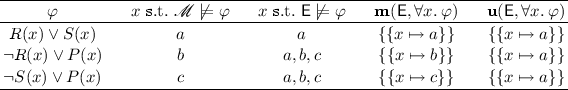The second and third columns show the sets of possible values of x that are considered with model-based and enumerative instantiation respectively, and the third and fourth columns show one possible selection. The instances corresponding to the three substitutions returned by enumerative instantiation $$R( a ) \vee S( a )$$, $$\lnot R( a ) \vee P( a )$$ and $$\lnot S( a ) \vee P( a )$$ when conjoined with $$\lnot P( a )$$ from $$\mathsf {E}$$ are unsatisfiable, whereas the instances produced by model-based instantiation do not suffice to show that $$\mathsf {E}$$ is unsatisfiable. Hence, the latter will consider an extension of $$\mathsf {E}$$ that satisfies the instances $$R( a ) \vee S( a )$$, $$\lnot R( b ) \vee P( b )$$ and $$\lnot S( c ) \vee P( c )$$ and guess another model for this extension.    $$\square$$

A key observation is that useful instantiations can be obscured by guesses made when constructing models $$\mathscr {M}$$. Here, since we decided $$R( a )^\mathscr {M}= \bot$$, the substitution $$\{ x \mapsto a \}$$ was not considered when applying model-based instantiation to the second quantified formula, and since $$S( a )^\mathscr {M}= \bot$$, the substitution $$\{ x \mapsto a \}$$ was not considered when applying it to the third. In implementations of model-based instantiation, certain values in models are chosen heuristically, leading to this behavior. This is done out of necessity, since determining whether there exists a model that satisfies quantified formulas, even for a fixed context, is a challenging problem.

On the other hand, the range of substitutions considered by enumerative instantiation in the previous example include all terms that correspond to instances that are not entailed by $$\mathsf {E}$$. The substitutions it considers are “minimally diverse”, that is, in the previous example they introduce new predicates on term a only, whereas model-based instantiation introduces new predicates on a, b and c. Reducing the number of new terms introduced by instantiations can have a significant positive impact on performance in practice. Furthermore, enumerative instantiation has the advantage that a term ordering allows fine-grained heuristics better suited for unsatisfiable problems, which we comment on in Sect. 4.1.

### Example 3

Consider the sets $$\mathsf {E}= \{ a \not \approx b,\, b \not \approx c,\, a \not \approx c \}$$ and $$\mathsf {Q}= \{ \forall x.\>P( x ) \}$$. For the input $$( \mathsf {E},\, \forall x.\> P( x ) )$$, model-based quantifier instantiation will first construct a model $$\mathscr {M}$$ for $$\mathsf {E}$$, where assume that $$P^\mathscr {M}= \lambda x.\> \top$$. It is easy to see $$\mathscr {M}\,\models \,\varphi \{ x \mapsto t \}$$ for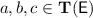, and hence it returns the empty set of substitutions, indicating that $$\mathsf {E}\cup \mathsf {Q}$$ is satisfiable. On the other hand, assume enumerative instantiation chooses the lexicographic extension of a term ordering $$\preceq$$ where $$a \prec b \prec c$$. Since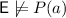and a is smaller than b and c according to $$\preceq$$, $$\mathbf{u}( \mathsf {E},\, P( x ) )$$ returns the set containing $$\{ x \mapsto a \}$$. Subsequently and for similar reasons, two more iterations of this strategy will be invoked, resulting in the instances P(b) and P(c) before it terminates with the empty set.    $$\square$$

In this example, model-based instantiation was able to terminate on the first iteration, since it guessed the correct interpretation for P, whereas enumerative instantiation considered substitutions mapping x to each ground term abc from $$\mathsf {E}$$. For this reason, model-based instantiation is typically better suited for satisfiable problems.

### 4.1 Implementing Enumerative Instantiation

We comment on several important details concerning the implementation of enumerative quantifier instantiation in the SMT solver CVC4.

Term Ordering. Given a term ordering $$\preceq$$, CVC4 considers the extension to tuples of terms such that:

\begin{aligned} \begin{array}{lcr} ( t_1, \ldots , t_n ) \prec ( s_1, \ldots , s_n ) &{} \text { if } &{} {\left\{ \begin{array}{ll} {\mathop {\max }\nolimits _{i=1}^n} t_i \prec {\mathop {\max }\nolimits _{i=1}^n} s_i, \text { or } \\ {\mathop {\max }\nolimits _{i=1}^n} t_i = {\mathop {\max }\nolimits _{i=1}^n} s_i \text { and } ( t_1, \ldots , t_n ) \prec _{\text {lex}} ( s_1, \ldots , s_n ) \end{array}\right. } \end{array} \end{aligned}

where $$\prec _{\text {lex}}$$ is the lexicographic extension of $$\prec$$. For example, if $$a \prec b \prec c$$, then we have that $$( a, a ) \prec ( a, b ) \prec ( b, a ) \prec ( b, b ) \prec ( a, c ) \prec ( c, b ) \prec ( c, c )$$. By this ordering, we consider substitutions involving c only after all combinations of substitutions involving a and b are considered. This choice is important since it leads to instantiations that introduce fewer terms, and are thus more likely to lead to conflicts at the ground level.

The underlying term ordering is determined dynamically based on the current set of assertions $$\mathsf {E}$$. At all times, we maintain a finite list of quantifier-free terms such that we have fixed the ordering $$t_1 \prec \ldots \prec t_n$$. Then, if all combinations of instantiations for $$t_1, \ldots , t_n$$ are currently entailed by $$\mathsf {E}$$, we choose a termthat is such that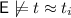for $$i = 1, \ldots , n$$ if one exists, and append it to our ordering so that $$t_n \prec t$$. The particular choice of t beyond this criteria is arbitrary. An experimental evaluation of more sophisticated term orderings, such as those inspired by first-order automated theorem proving  is the subject of future work.

Entailment Checks. For a set of ground equalities and disequalities $$\mathsf {E}$$, quantified formula $$\forall \bar{x}.\> \varphi$$ and substitution $$\{ \bar{x} \mapsto \bar{t} \}$$, CVC4 implements a two-layered method for checking whether the entailment $$\mathsf {E}\,\models \,\varphi \{ \bar{x} \mapsto \bar{t} \}$$ holds. First, we maintain a cache of instantiations that have already been returned on previous iterations. Hence if $$\mathsf {E}$$ satisfies a set of formulas containing $$\varphi \{ \bar{x} \mapsto \bar{s} \}$$, where $$\mathsf {E}\,\models \,\bar{t} \approx \bar{s}$$, then the entailment clearly holds.

Second, we use an incomplete and fast method for inferring when an entailment holds. We first compute from $$\mathsf {E}$$ congruence classes over. For each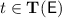, let $$[ t ]$$ be the representative of term t in this equivalence relation. For each function f, we use a term index data structure $$\mathscr {I}_{f}$$ that stores an entry of the form $$( [ t_1 ], \ldots , [ t_n ] ) \rightarrow [ f( t_1, \ldots , t_n ) ] \in \mathscr {I}_{f}$$ for each uninterpreted function application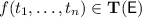. To check the entailment of $$\mathsf {E}\,\models \,\ell$$ where $$\ell$$ is a literal, we update $$\ell$$ based on the iterative process until a fixed point is reached:

1. 1.

Replace each constant t in $$\ell$$ with $$[ t ]$$.

2. 2.

Replace each function term $$f( t_1, \ldots , t_n )$$ in $$\ell$$ with s if $$( t_1, \ldots , t_n ) \rightarrow s \in \mathscr {I}_{f}$$.

3. 3.

If $$\ell$$ is $$t \approx t$$, replace it by $$\top$$.

4. 4.

If $$\ell$$ is $$t \not \approx s$$ and $$t' \not \approx s' \in \mathsf {E}$$ where $$[ t' ] = t$$ and $$[ s' ] = s$$, replace it by $$\top$$.

Then, if the resultant $$\psi$$ is $$\top$$, then the entailment holds. Although not shown here, the above process is extended in a straightforward way to handle Boolean structure, and also can be extended in the presence of other background theories in a straightforward way by incorporating theory-specific rewriting steps.

Restricting Enumeration Space. Enumerative instantiation can be refined further by noticing that only a subset of the set of terms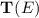will ever be relevant for showing unsatisfiability of a quantified formula. An approach in this spirit was used by Ge and de Moura , where decidable fragments were identified by noticing that the relevant domains of quantified formulas in these fragments are guaranteed to be finite. In that work, the relevant domain of a quantified formula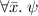is computed based on the terms in $$\mathsf {E}$$ and the structure of its body $$\psi$$. For example, t is in the relevant domain of function f for all ground terms f(t), the relevant domain of x for a quantified formula containing the term f(x) is equal to the relevant domain of f, and so on. A related approach is to use sort inference [8, 9, 22], to compute more precise sort information and thus decrease the number of possible instantiations.

### Example 4

Say $$\mathsf {E}\cup \mathsf {Q}= \{ a \not \approx b, f( a ) \approx c \} \cup \{ \forall x.\>P( f( x ) ) \}$$, where abcx are of sort $$\tau$$, f is a unary function $$\tau \rightarrow \tau$$, and P is a predicate on $$\tau$$. It can be shown that $$\mathsf {E}\cup \mathsf {Q}$$ is equivalent to $$\mathsf {E}^s \cup \mathsf {Q}^s =$$ $$\{ a_1 \not \approx b_1, f_{12}( a_1 ) \approx c_2 \} \cup \{ P_2( f_{12}( x_1 ) ) \}$$, where $$a_1, b_1$$, $$x_1$$ are of sort $$\tau _1$$, $$c_2$$ is of sort $$\tau _2$$, $$f_{12}$$ is of sort $$\tau _1 \rightarrow \tau _2$$, and $$P_2$$ is a predicate on $$\tau _2$$.    $$\square$$

Sorts can be inferred in this manner using a linear traversal on the input formula (for details, see for instance Sect. 4 of ). This technique narrows the set of terms considered by enumerative instantiation. In the above example, whereas enumerative instantiation for $$\mathsf {E}\cup \mathsf {Q}$$ might consider the substitutions $$\{ x \mapsto c \}$$ or $$\{ x \mapsto f( c ) \}$$, for $$\mathsf {E}^s \cup \mathsf {Q}^s$$ it would not consider $$\{ x_1 \mapsto c_2 \}$$ since their sorts are different, nor would it consider $$\{ x_1 \mapsto f_{12}( c_2 ) \}$$ since $$f_{12}( c_2 )$$ is not a well-sorted term. Moreover, the Herbrand universe of an inferred subsort may be finite when the universe of its parent sort is infinite. In the above example, the Herbrand universe of $$\tau _1$$ is $$\{ a_1,b_1 \}$$ and $$\tau _2$$ is $$\{ f_{12}( a_1 ), f_{12}( b_1 ), c_2 \}$$, whereas the Herbrand universe of $$\tau$$ is infinite.

Compound Strategies. Since the instantiation strategies from this section have their respective strengths and weaknesses, it is valuable to combine them. We consider two ways of combining strategies which we refer as priority instantiation and interleaved instantiation. For base strategies $$\mathbf{s_1}$$ and $$\mathbf{s_2}$$, priority instantiation ($$\mathbf{s_1};\mathbf{s_2}$$) first invokes $$\mathbf{s_1}$$. If this strategy returns a non-empty set of substitutions, it returns that set, otherwise it returns the instances returned by $$\mathbf{s_2}$$. On the other hand, interleaved instantiation ($$\mathbf {s_1}$$+$$\mathbf {s_2}$$) returns the union of the substitutions returned by the two strategies.

Enumerative instantiation is the most effective when used as a complement to heuristic strategies. In particular, we will see in the next section that the strategies c;e;u and c;e+u are the most effective strategies for unsatisfiable problems in CVC4.

## 5 Experiments

This section reports on our experimental evaluation of different strategies based on enumerative instantiation as implemented in the SMT solver CVC4.Footnote 2 We present an extensive analysis of enumerative instantiation and compare it with implementations of model-based instantiation on both unsatisfiable and satisfiable benchmarks. Experiments were performed on untyped first-order benchmarks from the TPTP library Footnote 3, version 6.4.0, and from SMT-LIB , as of October 2017, on logics having quantifiers and either uninterpreted functions or arrays. For the latter, we considered also logics containing other theories such as arithmetic and datatypes. Some benchmarks are solved by all considered configurations of solvers in less than 0.1 s. We discarded those $$25\,580$$ benchmarks. In total, $$42\,065$$ problems were selected, $$14\,731$$ from TPTP and $$27\,334$$ from SMT-LIB. All results were produced on StarExec , a public execution service for running comparative evaluations of solvers, with a timeout of 300 s.

We follow the convention in Sect. 4 for identifying configurations based on their instantiation strategy. All configurations of CVC4 use conflict-based instantiation [5, 28] with highest priority, so we omit the prefix “c;” from the names of CVC4 configurations e.g. e+u in fact means c;e+u. Sort inference, as discussed in Sect. 4.1, is also used by all configurations of CVC4.

### 5.1 Impact of Enumerative Instantiation in CVC4

In this section, we highlight the impact of enumerative instantiation in CVC4 for unsatisfiable benchmarks. Where applicable, we contrast the difference in the impact of enumerative instantiation and model-based instantiation on the performance of CVC4 on unsatisfiable benchmarks.Footnote 4

The comparison of various instantiation strategies supported by CVC4 is summarized in Fig. 3. In the table, each row is dedicated to a library and logic. SMT-LIB is shown in more granularity than TPTP to highlight comparisons of individual strategies. The first column identifies the subset and the second shows its total number of benchmarks. The next seven columns show the number of benchmarks found to be unsatisfiable by each configuration. The last three columns show the results of virtual portfolio solvers, with uport combining e, u, e;u, and e+u; and mport combining e, m, e;m, and e+m; while port combines all seven configurations.

First, we can see that u outperforms m, as it solves $$3\,043$$ more benchmarks overall. While this is not close to the performance of E-matching (e), it should be noted that u is highly orthogonal to e, solving $$1\,737$$ benchmarks that could not be solved by eFootnote 5. Combining e with either u or m, using either priority or interleaving instantiation, leads to significant gains in performance. Overall the best configuration is e+u, that is, the interleaving of enumerative instantiation and E-matching, which solves $$20\,535$$ benchmarks, that is, 253 more than its counterpart e+m interleaving model-based instantiation and E-matching, and $$1\,295$$ more than E-matching alone. In the UFLIA logic, the enumerative techniques are specially effective in comparison with the model-based ones. In particular, they enable CVC4 to solve previously intractable problems, e.g. the family “sexpr” with 32 problems. These are notoriously hard problems involving the verification of C# programs using Spec# . Z3 can solve 31 of them thanks to its advanced optimizations of E-matching . CVC4 previously could solve at most 16 using techniques combining e and m, but u alone could solve 27, and all of 32 are solved by e+u. Another example is the family “vcc-havoc” in UFNIA, stemming from the verification of concurrent C with VCC . The strategy e+u solves 940 out of 984 problems, outperforming e and its combinations with m, which solve at most 860 problemsFootnote 6.

The portfolio columns of the table in Fig. 3 highlight the improvement due to enumerative instantiation for CVC4 on the number of solved problems: there are 712 more problems overall solved when adding enumerative instantiation in the strategies (see columns mport and port). The cactus plot of Fig. 3 shows that while the priority strategies are initially quicker, the interleaving ones scale better, solving more hard problems than their priority counterparts. Overall, we conclude that in addition to being much simpler to implementFootnote 7 instantiation strategies that combine E-matching with enumerative instantiation in CVC4 have a noticeable advantage over those that combine E-matching with model-based instantiation on unsatisfiable problems.

### 5.2 Comparison Against Other SMT Solvers

In this section, we compare our implementation of enumerative instantiation in CVC4 against another state-of-the-art SMT solver: Z3  (version 4.5.1) which, like CVC4, also relies on E-matching instantiation for handling unsatisfiable problems. Before making the comparison, we first summarize the main differences between Z3 and CVC4 here. Z3 uses several optimizations for E-matching that are not implemented in CVC4, including the use of code trees and techniques for applying instantiation incrementally during the CDCL($$\mathscr {T}$$) search (see Sect. 5 of ). It also implements techniques for removing previously considered instantiations from its set of known clauses (see Sect. 7 of ). The main advantage of CVC4 with respect to Z3 is its use of conflict-based instantiation $$\mathbf{c}$$ , which is enabled by default in all strategies we considered. It also supports interleaved instantiation strategies as described in Sect. 4.1, whereas Z3 does not. In addition to these differences, Z3 implements model-based instantiation m as described in , whereas CVC4 implements model-based instantiation as described in . Finally, CVC4 implements enumerative instantiation as described in this paper, which we compare as an alternative to these implementations.

Figure 4 summarizes the performance of Z3 on our benchmark set. First, like CVC4, using model-based instantiation to complement E-matching leads to significant gains in Z3, as z3 e;m solves a total of 1731 more benchmarks than solved by E-matching alone z3 e. In comparison with CVC4, the configuration z3 e outperforms e in the logics with non-linear arithmetic and other theories, while e is better in the others. Finally, Z3’s implementation of model-based quantifier instantiation by itself z3 m is not effective for unsatisfiable benchmarks, solving only 8951 overall.

To further compare Z3 and CVC4, the third column from the left is the number of benchmarks solved by CVC4’s E-matching strategy (e), which we gave in Fig. 3. The second to last column uport-i gives the number of benchmarks solved by at least one of u, e, or e;u in CVC4, where we intentionally omit the interleaved strategy e+u, since Z3 does not support a similar strategy. The column mport-i is computed similarly. We compare these with the fifth column, z3 mport-i, i.e. the number of benchmarks solved by either z3 m, z3 e or z3 e;m. A comparison of these is given in the cactus plot of Fig. 4. We can see that due to Z3’s highly optimized implementations, z3 mport-i solves the highest number of problems in less than one second (around 13000), whereas the portfolio strategies of CVC4 solve more for larger timeouts. Overall, the best portfolio strategy is enumerative instantiation in CVC4, which solves a total of 21305 unsatisfiable benchmarks overall, which is 1965 more benchmarks than z3 mport-i, and 470 more benchmarks than mport-i. We thus conclude that the use of enumerative instantiation when paired with E-matching and conflict-based instantiation in CVC4 improves the state-of-the-art of instantiation-based SMT solvers for unsatisfiable benchmarks.

Comparison with Automated Theorem Provers. Automated theorem provers like Vampire  and E  use substantially different techniques based on superposition, hence we do not provide an extensive comparison here. However, we do remark that the gains provided by enumerative instantiation were one of the main reasons for CVC4 being more competitive in the 2017 CASC competition of automatic theorem provers . CVC4 placed third in the category with unsatisfiable problems on the empty theory, as in previous years, behind superposition-based theorem provers Vampire and E, which implement complete strategies. There was, however, a $$23\%$$ reduction in the number of problems that E solves and CVC4 does not, w.r.t. the previous competition, reducing the gap between the two systems.

Satisfiable Benchmarks. For satisfiable benchmarksFootnote 8, m solves 1350 benchmarks across all theories. As expected, this is much higher than the number solved by u, which solves 510 benchmarks, all from the empty theory. Nevertheless, there are 13 satisfiable problems solved by u and not by m, which shows that enumerative instantiation has some orthogonality on satisfiable benchmarks as well. We conclude that enumeration not only has superior performance to MBQI on unsatisfiable benchmarks, but also can be an alternative for satisfiable benchmarks in the empty theory.

### 5.3 Artifact

We have produced an artifact  to reproduce the experimental results presented in this paper. The artifact contains the binaries of the SMT solvers CVC4 and Z3, the benchmarks on which they were evaluated, and the running scripts for each configuration evaluated. Detailed instructions are given to perform tests on the various benchmark families with all configurations within the time limits, as well as for retrieving the respective results in CSV format. The artifact has been tested in the virtual machine available at .

## 6 Conclusion

We have presented a strengthening of the Herbrand Theorem, and used it to devise an efficient technique for enumerative instantiation. The implementation of this technique in the state-of-the-art SMT solver CVC4 increases its success rate and outperforms existing implementations of MBQI on unsatisfiable problems with quantified formulas. Given its relatively simple implementation, this technique is well poised as an alternative to MBQI for being integrated in an instantiation based SMT solver to achieve completeness in first-order logic with the empty theory and equality, as well as perform improvements also when theories are considered.

Future work includes further restricting the enumeration space, for instance with ordering criteria in the spirit of resolution-based theorem proving . Another direction is lifting the techniques seen here to reasoning in higher-order logic. To handle quantification over functions it is often necessary to enumerate expressions, and so performing such an enumeration in a principled manner is paramount for this domain. Techniques from syntax-guided function synthesis  could be combined with enumerative instantiation to pursue this goal.

Data Availability Statement and Acknowledgments. The datasets generated and analyzed during the current study are available in the figshare repository: https://doi.org/10.6084/m9.figshare.5917384.v1.

This work was partially funded by the National Science Foundation under Award 1656926, by the H2020-FETOPEN-2016-2017-CSA project SC$$^\mathsf {2}$$ (712689), and by the European Research Council (ERC) starting grant Matryoshka (713999). We would like to thank the anonymous reviewers for their comments. We are grateful to Jasmin C. Blanchette for discussions, encouragements and financial support through his ERC grant.